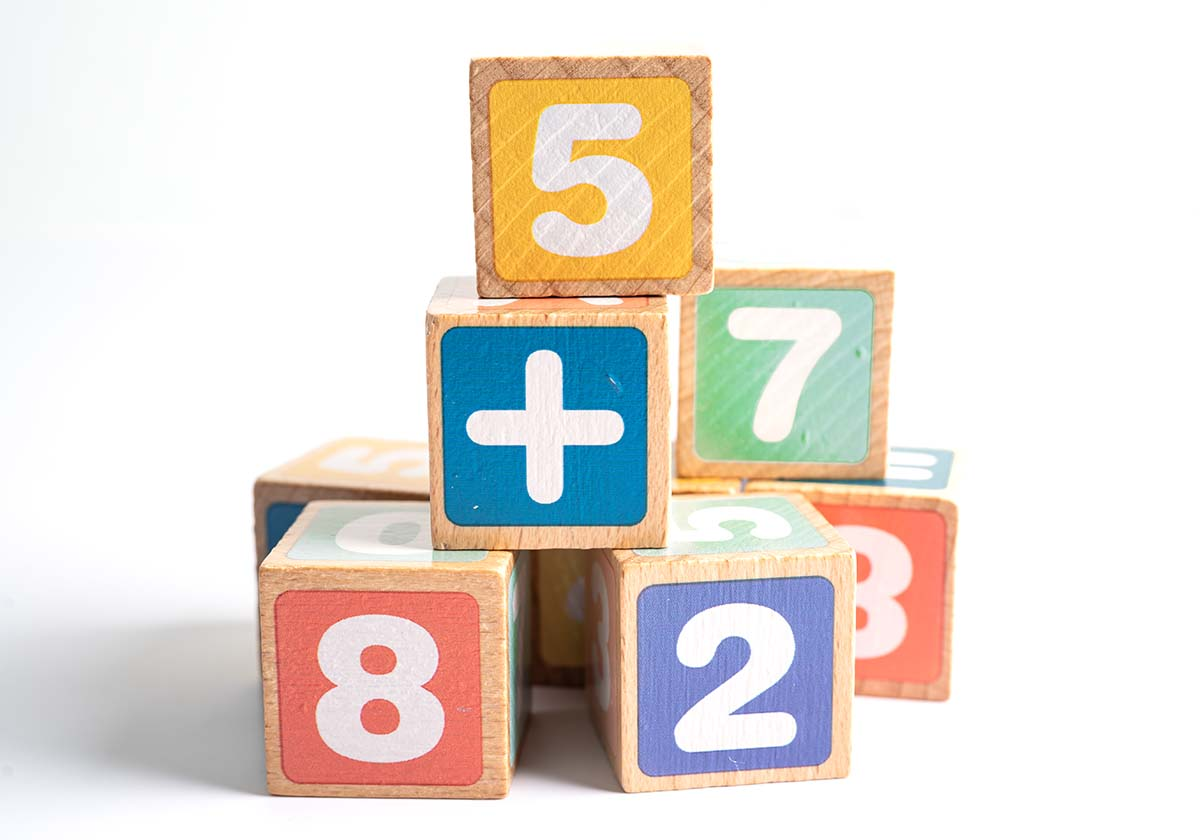Math symbols are used in all levels of math. However, children are sometimes confused about math symbols’ meaning and how to use them.  This article shows the meaning behind four common math symbols children will encounter. By learning these symbols, children will be more comfortable in solving various math problems at school and in real life!One of the first symbols children learn is the math (+) symbol, also known as the addition or “plus” symbol. These two crossed lines means that numbers need to be added to the problem. An example of a math problem using this symbol is 6+2. You can also read it as “six plus two”. The answer is found by adding 2 to 6, or counting on 2 numbers after 6, which equals to the number 8. You can also visualize this problem in a real-life setting. For instance, an alternative question is “how many fruits are you buying if you buy 6 apples and 2 bananas?”. The answer’s the same. When we add 6 apples and 2 bananas, we get 8 fruits in all!

### Subtraction (-)

The math (-) symbol, known as the subtraction or “minus” symbol, is also commonly used. The horizontal dash line means to subtract or reduce a certain number from the problem. A simple math problem using this symbol is 10-3, which can also be read as “ten minus three”. This problem requires counting back 3 numbers from the number 10, which equals to the number 7. Another visualization of the problem can be “if you have 10 pencils and 3 are taken from you, how many pencils do you have left?”. You guessed it, this problem is the same as taking away 3 from 10 and that gives us 7 pencils!

### Multiplication (x)

Another common math symbol is the multiplication symbol (x), often called “times” in real life. This symbol, which also looks like the letter “x”, means to multiply two sets of numbers together.  A math problem example using multiplication is 8×4. You can also read this as “eight times four”. In this case, the number 8 is multiplied by 4, which results in the number 24. This problem can also be visualized in real-life as “if 4 children receive 8 books each, how many books are there in total?”. If you add 4 groups of 8, you’ll also get 24!

### Division (÷)

One of the final set of symbols in math is the division symbol (÷). This symbol means to divide one number by another number. A math problem example using division is 10÷5. You can also read this as “ten divided by five”. If we divide the number 10 into 5 parts, we get an even answer of 2. Another example of this problem will be “if we give out a total of 10 snacks to 5 children, how many snacks does one child receive?”. In this case, each child receives 2 snacks!

### Conclusion

Understanding math symbols’ names and meanings will definitely be a big help to children! If they are still uncertain, they can use this article as a guide and copy paste any math symbols if need be. Children can also familiarise themselves with these symbols by solving daily questions on Practicle’s gaming app. Not only can they learn math at their own pace, they can also have fun while doing it!

### One Comment

•annisa says:

Simple but it’s something that every child should know. Thank you!